# GMAT Math : DSQ: Calculating the slope of a perpendicular line

## Example Questions

### Example Question #91 : Coordinate Geometry

Calculate the slope of a line perpendicular to line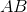.

1. Linepasses through points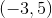and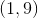.
2. The equation for lineis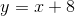.

Statement 2 alone is sufficient, but statement 1 alone is not sufficient to answer the question.

Statements 1 and 2 are not sufficient, and additional data is needed to answer the question.

Statement 1 alone is sufficient, but statement 2 alone is not sufficient to answer the question.

Each statement alone is sufficient to answer the question.

Both statements taken together are sufficient to answer the question, but neither statement alone is sufficient.

Each statement alone is sufficient to answer the question.

Explanation:

Statement 1: We can use the points provided to find the slope of line AB.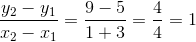Since the slope we're being asked for is of a line perpendicular to line AB, their slopes are inverses of each other.

The slope of our line is then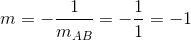Statement 2: Since we're provided with the line's equation, we just need to look for the slope.Whereis the slope andis the y-intercept.

In this case, we haveso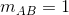. Because our line is perpendicular to line AB, the slope we're looking for is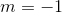### Example Question #92 : Coordinate Geometry

Find the slope of a line perpendicular to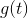.

I)passes through the points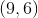and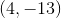.

II)does not pass through the origin.

Either statement is sufficient to answer the question.

Both statements are needed to answer the question.

Statement I is sufficient to answer the question, but statement II is not sufficient to answer the question.

Statement II is sufficient to answer the question, but statement I is not sufficient to answer the question.

Statement I is sufficient to answer the question, but statement II is not sufficient to answer the question.

Explanation:

Find the slope of a line perpendicular to g(t)

I) g(t) passes through the points (9,6) and (4,-13)

II) g(t) does not pass through the origin

Perpendicular lines have opposite reciprocal slopes. For instance: a line with a slope ofwould be perpendicular to a line with slope of.

To find the slope of a line, we just need two points.

I) Gives us two points on g(t). We could find the slope of g(t) and then the slope of any line perpendicular to g(t).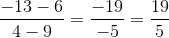So the slope of a line perpendicular to g(t) is equal to: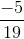II) Is irrelevant or at least not helpful.

### Example Question #93 : Coordinate Geometry

Consider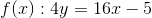and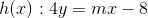.

Find the slope of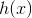.

I)passes through the point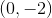.

II)is perpendicular to.

Either statement alone is sufficient to answer the question.

Statement II is sufficient to answer the question, but Statement I is not sufficient to answer the question.

Both statements together are needed to answer the question.

Statement I is sufficient to answer the question, but Statement II is not sufficient to answer the question.

Statement II is sufficient to answer the question, but Statement I is not sufficient to answer the question.

Explanation:

We are given a line, f(x), and asked to find the slope of another line, h(x).

I) Gives a point on h(x). We could plug in the point and solve for our slope. When we do this since x=0 we are unable to find the value for our slope. Therefore, statement I is not sufficient to solve the question.

II) Tells us the two lines are perpendicular. Take the opposite reciprocal of the slope of f(x) to find the slope of h(x).

Therefore,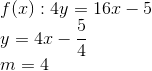and thus the slope of h(x) will be,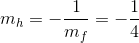.

Statement II is sufficient to answer the question.

Tired of practice problems?

Try live online GMAT Math prep today.